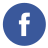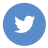### Java Basic Datatypes

java

In Java, there are two categories of data types: primitive types and reference types.

Primitive types are the basic data types in Java and include:

`boolean`: represents a boolean value (true or false)

`char`: represents a single Unicode character

`byte`: represents an 8-bit signed integer

`short`: represents a 16-bit signed integer

`int`: represents a 32-bit signed integer

`long`: represents a 64-bit signed integer

`float`: represents a single-precision floating-point number

`double`: represents a double-precision floating-point number

Here are some examples of how to declare and initialize variables of primitive types:

``````boolean flag = true;
char ch = 'A';
byte b = 100;
short s = 10000;
int i = 100000;
long l = 1000000L;
float f = 3.14f;
double d = 3.14;``````

Reference types, on the other hand, refer to objects in Java. All classes, arrays, and interface types are reference types. Reference types are created using the `new` operator and are stored on the heap.

Here is an example of how to declare and initialize a reference type variable:

``String str = new String("Hello");``

In this example, the `str` variable is of type `String` and refers to a new `String` object with the value “Hello”.